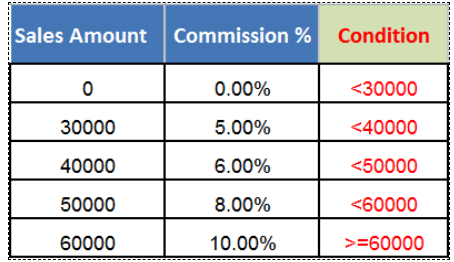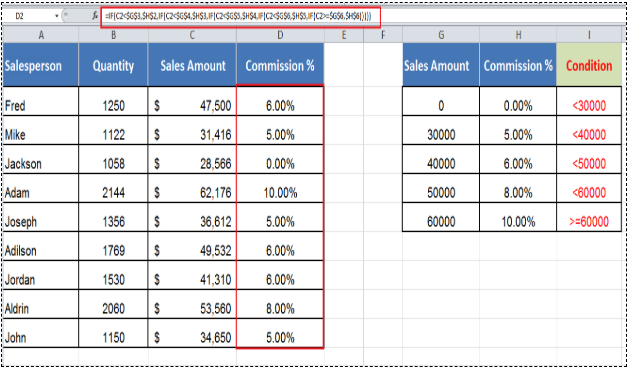Get instant live expert help with Excel or Google Sheets“My Excelchat expert helped me in less than 20 minutes, saving me what would have been 5 hours of work!”

#### Post your problem and you’ll get expert help in seconds.

Your message must be at least 40 characters
Our professional experts are available now. Your privacy is guaranteed.

# Learn How to Use Nested IF Statements in Excel

As per syntax, a basic IF statement handles one logical test, and based on its TRUE and FALSE values, it specifies one value_if_true argument’s value and one value_if_false argument’s value. But there are some cases where there are multiple logical tests and multiple value_if_true arguments’ values, having a separate value in each TRUE case. For that purpose you need to create multiple, or nested, IF statements for each logical test and its value_if_true argument as per the basic syntax of the IF formula.

## Using nested IF statements in Excel

Nested means to combine two formulas together, one inside the other and outer formula controls the result of the inner formula, like;

`=DAY(TODAY())`

In this nested formula example, the TODAY function returns today’s date inside DAY function and outer DAY function returns the day of the month for date returned by TODAY function.

In the same fashion, two or more IF statements can be nested together, one inside the other, but the only difference between other nested formulas and nested IF formulas is that outer IF formula runs first in IF statements.

First look at the syntax of two nested IF statements and a brief explanation.

`IF(logical_test1, [value_if_true1], IF(logical_test2, [value_if_true2], [value_if_false]))`

Here, in this nested IF statements you can see two IF statements combined together, one insider the other. For a better understanding of nested IF statements’ syntax and pattern, each IF statement is presented in a separate font color. The 2nd IF statement starts followed by the value_if_true argument of 1st IF statement, and so on.

In the last IF statement, you can optionally supply value_if_false argument and close the nested IF formula by the number of brackets equal to the number of IF statements nested in the formula. For example, if you have nested three IF statements in a formula, then it must contain three closing brackets at the end.

The trick is that if outer logical_test argument (first logical test) comes TRUE, then it returns first value_if_true argument’s value, and it ignores the inner IF formulas. If outer IF formula logical_test argument comes FALSE then inner IF formulas are run.

As a general information regarding nested IF statements formula, you can nest or combine 7 IF statements in one formula till MS Excel 2007. But in MS Excel 2010 and later versions, you can nest up to 64 IF statements.

Generally, nested IF statements are used for various scales, like grade structure, commission structure, tax structure and so on… Sometimes too large of a scale structure results in very complicated nested IF statements, so it is very important to use nested IF statement wisely otherwise it gets complicated to understand easily.

In this example, you will learn how to create multiple nested IF statements in a single formula. Suppose you have sales data from a company for various salespersons. You have a sales commission structure based on sales amount as given below, and you need to assign a commission % as per structure using nested IF statements approach.In the above screen shot you have a commission structure based on sales amounts and for your better understanding, we have also setup conditions or logical tests used in nested IF statements in this example.

Now by using each condition or logical test in each IF statement, you will nest all the five IF statements in a single formula as follows;

`=IF(C2<30000,0%,IF(C2<40000,5%,IF(C2<50000,6%,IF(C2<60000,8%,IF(C2>=60000,10%)))))`

OR

`=IF(C2<\$G\$3,\$H\$2,IF(C2<\$G\$4,\$H\$3,IF(C2<\$G\$5,\$H\$4,IF(C2<\$G\$6,\$H\$5,IF(C2>=\$G\$6,\$H\$6)))))`Still need some help with Excel formatting or have other questions about Excel? Connect with a live Excel expert here for some 1 on 1 help. Your first session is always free.

Are you still looking for help with the IF function? View our comprehensive round-up of IF function tutorials here.

### Did this post not answer your question? Get a solution from connecting with the expert.Another blog reader asked this question today on Excelchat:
Solution examplesI have a list or people who are ranked in numbers from 3 to 6 I need to recognize the contents of each multiple cells and create an equivalent letter. for that value into another column. e.g. Cell E1 = 3 to show in new cell that row (H1) the letter "C" I have multiple rows with different values in column "E" Can this be done?
Solved by I. J. in 30 minscan you teach me the steps to create a nested if function, and to nest an AND function inside of an IF function?
Solved by A. Q. in 22 minsI have a spreadsheet where we track deadlines for questionnaire completions. This formula is currently set up like this: =IF(AND(ISBLANK(L2),ISBLANK(S2)),IF(TODAY()>=(K2),"Closed",IF(TODAY()<(J2),IF(TODAY()<(F2),"Not open","On-time"),"Late")),"Complete") I would like to add a condition so that if today is two weeks away from the deadline or closer, it shows up as "nearing deadline"
Solved by S. U. in 24 minsNEED A FORMULA TO SHOW THE FOLLOWING: IF (Q3) IS AFTER TODAY & (R3) IS BLANK,THEN "BEHIND SCHEDULE". IF (R3) IS POPULATED THEN "COMPLETE". IF (Q3) IS
Solved by E. U. in 42 minsCould you help me by explaining the IF function?
Solved by G. L. in 24 mins## Subscribe to Excelchat.coAnother blog reader asked this question today on Excelchat: